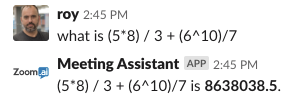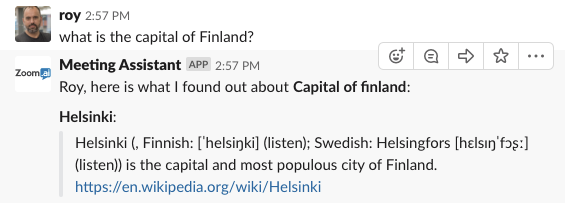### Math Questions

```what is 2+2?<br>what is (5*8) / 3 + (6^10)/7<br>what is sin(45 deg) ^ 2<br>what is sqrt(-4)
```### Unit Conversation Questions

```what is 32F in C?<br>what is 1000m in inches?<br>what is 1 tablespoon in ml
```### Wikipedia Questions

You can ask any question you want and CalendarHero will first search your configured Knowledge Base.  If no response is found, then it will search Wikipedia.org for an answer.

```what is the capital of Finland?<br>what is the tardis?
```